Courses

# Test Level 1: Electric Charges And Fields

## 30 Questions MCQ Test Physics Class 12 | Test Level 1: Electric Charges And Fields

Description
This mock test of Test Level 1: Electric Charges And Fields for Class 12 helps you for every Class 12 entrance exam. This contains 30 Multiple Choice Questions for Class 12 Test Level 1: Electric Charges And Fields (mcq) to study with solutions a complete question bank. The solved questions answers in this Test Level 1: Electric Charges And Fields quiz give you a good mix of easy questions and tough questions. Class 12 students definitely take this Test Level 1: Electric Charges And Fields exercise for a better result in the exam. You can find other Test Level 1: Electric Charges And Fields extra questions, long questions & short questions for Class 12 on EduRev as well by searching above.
QUESTION: 1

### Which of the following methods can be used to charge a metal sphere positively without touching it . Select the best

Solution:

This can be possible by induction, if a negative charge rod is placed near the sphere (at a distance not in touch) than it attracts positive charge inside the sphere towards its side and the negative charges collected on other side and when the surface of sphere connected to earth than the negative charge goes to earth and the sphere remain positively charged.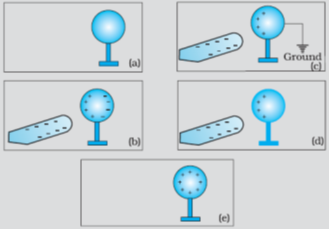QUESTION: 2

### Electric field lines can be said to be

Solution:

Electric Field Lines can be defined as a curve which shows direction of electric field, when we draw tangent at its point. The concept of electric field was proposed by Michael Faraday, in the 19th century. He always thought that electric field lines can be used to describe and interpret the invisible electric field. Instead of using complex vector diagram every time, This pictorial representation or form is called electric field lines.Electric field lines can be used to describe electric field around a system of charges in a better way.

QUESTION: 3

### When a negatively charged conductor is connected to earth,

Solution:

After earthing a positively charged conductor electrons flow from earth to conductor and if a negatively charged conductor is earthed then electrons flows from conductor to earth.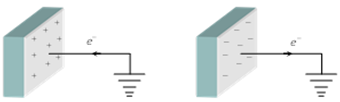QUESTION: 4

A point charge of 2.0 μC is at the centre of a cubic Gaussian surface 9.0 cm on edge. What is the net electric flux through the surface?

Solution: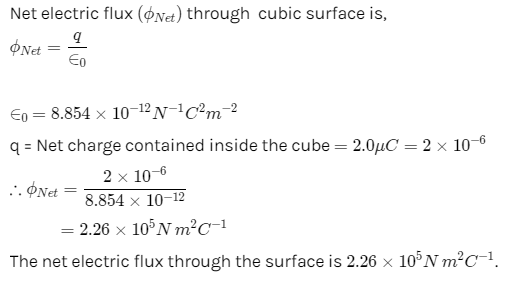QUESTION: 5

Two equal positive charges q1 = q2 = 2.0 μC. μC are located at x = 0, y =0.3 and x =0 and y = -0.3 m respectively. What are the magnitude and direction of the total electric force (expressed in Newton and degrees counter clockwise w.r.t x - axis) that q1 and q2 exert on a third charge Q = 4.0 μC.  μC at x =0.4 and y = 0 m

Solution:

Solution :

The correct option is Option A.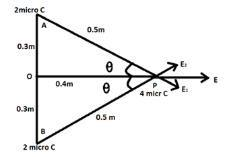Side AP = √(0.3² + 0.4²) = 0.5m

Electric Field due to charge at A on point P = Electric Field due to charge at B on E1 = E2 = q / 4or²

Due to charge at B on = 2 × 10-6 (9 × 10⁹) / 0.5²  at P

Point P, E = E1cosθ + E2cosθ = 2 × 72 × 10³ N/C

So, force due to this field on charge P

F = qE = 4 × 10^-6 × 115.2 × 10³ F = 0.46N

Since this force in the direction of positive x axis. So angle = 0°

QUESTION: 6

If 109electrons move out of a body to another body every second, how much time is approximately required to get a total charge of 1 C on the other body?

Solution: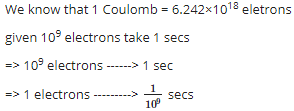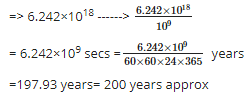QUESTION: 7

At any point on S on an electric field line

Solution:

When a tangent is drawn at any point on field line then that tangent gives the direction of electric field at that point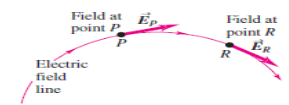QUESTION: 8

A point charge causes an electric flux of −1.0×103Nm2/C to pass through a spherical Gaussian surface of 10.0 cm radius centred on the charge.
(a) If the radius of the Gaussian surface were doubled, how much flux would pass through the surface?
(b) What is the value of the point charge?

Solution:

Electric flux is given by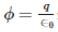since amount of charge not depends on size and shape so by making radius <  double the amount of charge remain same so electric flux remain same.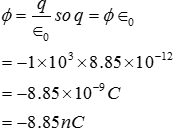QUESTION: 9

Positive and negative point charges of equal magnitude are kept at ( 0,0,a/2) and (0,0,-a/2) respectively. The work done by the electric field when another positive point charge is moved from (-a,0,0) to (0,a,0) is

Solution: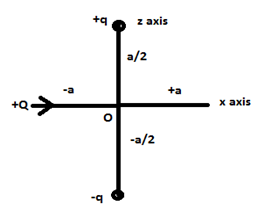+Q charge is moving on equatorial line of dipole as shown in figure, since on equatorial line V=0 So W=0

QUESTION: 10

Electric charge

Solution:

Every matter is made up of atoms which contains protons, electrons and neutrons.

Protons are +ve charged particles and electrons are - ve charged particles.

Because of electric charge these particles experience a force in electrical fields.

QUESTION: 11

An electric dipole is

Solution:

An electric dipole is a separation of positive and negative charges. The simplest example of this is a pair of electric charges of equal magnitude but opposite sign, separated by some (usually small) distance. A permanent electric dipole is called an electret.

QUESTION: 12

A hollow spherical conductor of radius 2m carries a charge of 500 μμ C. Then electric field strength at its surface is

Solution:

E = q/4πE(0)r^2

= [(9 * 10^9) (500 * 10^-6)]/4

= 1125 * 10^3

=1.125 * 10^6 N/C

QUESTION: 13

For forming an electric dipole between two point charges separated by a small distance, the two point charges are of

Solution:
QUESTION: 14

Three concentric metallic spherical shells of radii R, 2R, 3R, are given charges Q1, Q2, Q3, respectively. It is found that the surface charge densities on the outer surfaces of the shells are equal. Then, the ratio of the charges given to the shells, Q1: Q2: Q3, is

Solution: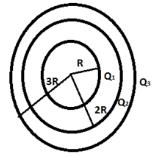Since the charge goes to outer surface when given inside so the charges on concentri shells are respectively.

Q1, Q1 + Q2, Q+ Q2 + Q3. Sine their charge densities are equal so σ1 = σ2 = σ3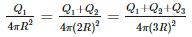So Q1 = 3Q2 = 5Q3 so 1 : 3 : 5

QUESTION: 15

The amount of work done in moving a charge from one point to another along an equipotential line or surface charge is

Solution:

Since Potential difference between two points in equipotential surfaces is zero , the work done between two points in equipotential surface is also zero .

QUESTION: 16

An electric field can deflect

Solution:

Only alpha rays are moving with small velocity and having charge so they will be affected by electric field.

QUESTION: 17

A tennis ball which has been covered with charges is suspended by a thread so that it hangs between two metal plates. One plate is earthed, while other is attracted to a high voltage generator. The ball

Solution:

The plate which connected to high voltage generator induces negative charge on ball which causes attraction. When the ball strikes the positive plate, charge distribution again takes place that is the bass becomes positive and repulsion takes place. When it strikes the plate which connected to earth than its charge goes to earth and again it will be attracted towards positive plate. Hence the ball swings backward and forward hitting each plate in turn.

QUESTION: 18

Under the influence of the coulomb field of charge +Q, a charge −q is moving around it in an elliptical orbit. Find out the correct statement(s).

Solution:

Since the charge –q is moving in elliptical orbit so to make its motion stable the total angular momentum of the charge is constant since it experience a centripetal force from the charge +Q so it follow the motion as the motion of earth around sun.

QUESTION: 19

Conservation of charges in tribo electric charging

Solution:

The triboelectric effect (also known as triboelectric charging) is a type of contact electrification in which certain materials become electrically charged after they come into frictional contact with a different material. Rubbing glass with fur, or a plastic comb through the hair, can build up triboelectricity.When we charge something with static electricity, no electrons are made or destroyed. No new protons appear or disappear. Electrons are just moved from one place to another. The net, or total, electric charge stays the same. This is called the principle of conservation of charge.If a glass rod is rubbed with silk, some electrons moves from glass rod to silk no new charge is generated or destroyed.

QUESTION: 20

Consider a neutral conducting sphere. A positive point charge is placed outside the sphere. The net charge on the sphere is then,

Solution:

If a charge q is placed outside than the electric field lines incident on the conducting sphere , if some charge is developed due to thee lines than opposite surface becomes oppositely charge and the total charge becomes zero.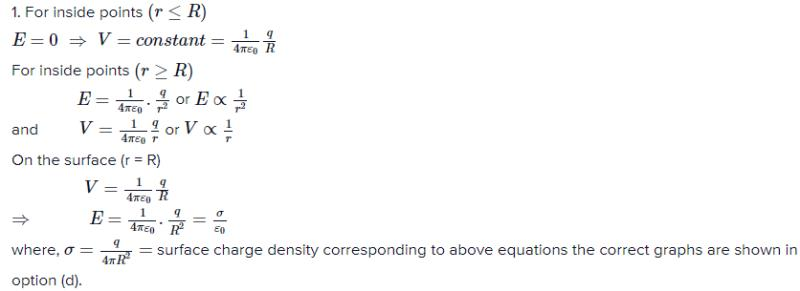QUESTION: 21

A point charge Q is moved along a circular path around another fixed point charge The work done is zero

Solution:

Since that circular path behave as equipotential surface so work done is always zero.

QUESTION: 22

Two positive charges

Solution:

Acc to coulomb's law unlike charges always attract each other while like charges always repel each other so between two positive charges repulsion is working.

QUESTION: 23

Two insulated charged copper spheres A and B have their centres separated by a distance of 50 cm. What is the mutual force of electrostatic repulsion if the charge on each is 6.5×10−7C ? The radii of A and B are negligible compared to the distance of separation.

Solution: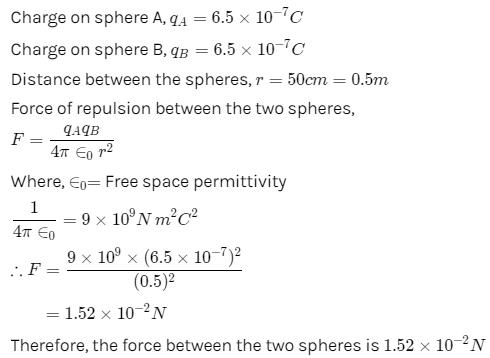QUESTION: 24

Ionization of a neutral atom is the

Solution:

It is not possible to remove or add protons to atom, but electron can be added or removed by an atom easily so charge can be developed on an atom by removing or adding electrons, by adding electrons it becomes negatively charged ,by removing electrons it becomes positively charged.

QUESTION: 25

A long, hollow conducting cylinder is kept coaxially inside another long, hollow conducting cylinder of larger radius. Both the cylinders are initially electrically neutral.

Solution:

When the charge is given to inner cylinder than there is an electric field is produced between cylinders which is given by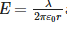and due to this a potential difference is developed between two cylinders.

QUESTION: 26

A charge q is placed at the center of the line joining two equal charges Q. The system of the three charges will be in equilibrium if q is equal to

Solution: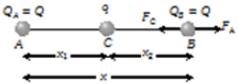Suppose in the following figure, equilibrium of charge B is considered. Hence for its equilibrium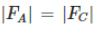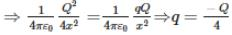QUESTION: 27

electrostatic force is

Solution:

Electrostatics means charges at rest,or relatively at rest so this force can be applicable only when charge produce electric field which is constant which is possible only when charges at rest or relatively at rest.

QUESTION: 28

Two charges A = -2.50 μC  and B = 6.0 μC are at a distance of 1 meter from each other. Distance from A at which the electric field is zero in meters is

Solution: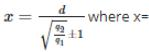distance of null point from small charge, d=distance between two charges, q2=bigger charge, q1=small charge. +1 for like charges and -1 for unlike chargesx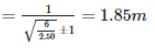QUESTION: 29

Find the electric field inside the sphere which carries a charge density proportional to the distance from the origin
ρ = kr

Solution:

∮E.ds=Q/ε0
​⇒Q=∫ρV​
ρV​=αr is given where r is radius
E.4πr2=(​ρV​(4/3)​πr3)/ε0
​E=​αr2​/3ε0

QUESTION: 30

Conductors are materials

Solution:

Conductors are materials that permit electrons to flow freely from particle to particle. An object made of a conducting material will permit charge to be transferred across the entire surface of the object.In conductors some electrons are unpaired so these electrons can move freely.